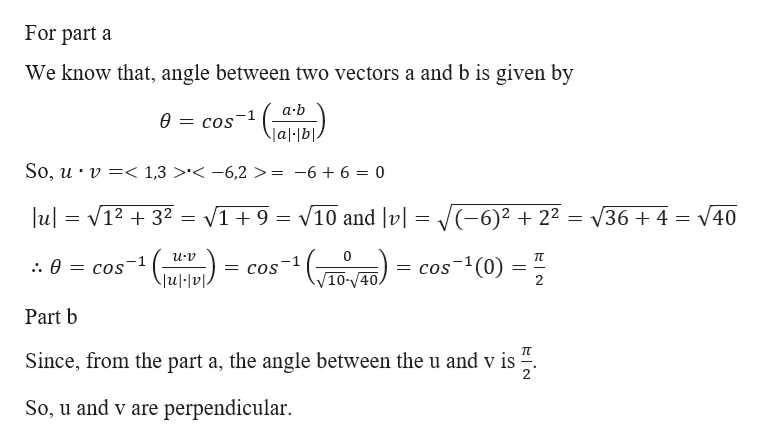# Let u=〈1,3〉and let v=〈−6,2〉(a) Find the angle between u and v(b) Are u and v perpendicular?

Question
18 views

Let u=〈1,3〉and let v=〈−6,2〉

(a) Find the angle between u and v

(b) Are u and v perpendicular?

check_circle

Step 1

Given,

Step 2

For part a and...help_outlineImage TranscriptioncloseFor part a We know that, angle between two vectors a and b is given by a.b Ө — cos" Jal bl. So, u v =< 1,3 '< -6,2 > = -6 + 6 = 0 u 1232 V19 10 and v|= 6)2 22= V36 + 4 = V40 0 TT -1 = COS = cos(0) .. Ө — сos 10-40. Jul|v1. Part b п Since, from the part a, the angle between the u and v is So, u and v are perpendicular. fullscreen

### Want to see the full answer?

See Solution

#### Want to see this answer and more?

Solutions are written by subject experts who are available 24/7. Questions are typically answered within 1 hour.*

See Solution
*Response times may vary by subject and question.
Tagged in

### Vectors